Peter and Franta threw to the basket. Each had 20 attempts. Peter scored thirteen and twelve Franta. Give them success in percentage.

p(P) =  65 %
p(F) =  60 %

### Step-by-step explanation:We will be pleased if You send us any improvements to this math problem. Thank you!Tips to related online calculators
Need help to calculate sum, simplify or multiply fractions? Try our fraction calculator.
Would you like to compute count of combinations?

## Related math problems and questions:

• The Chemistry testThe Chemistry test contained 8 questions, each with 3 points. Peter scored 21 points. How many percent did Peter write a test?
• Prime numberJan wrote any number from 1 to 20. What is the probability that he wrote the prime number?
• PediatricianPediatrician this month of 20 working days takes 8 days holidays. What is the probability that on Monday it will be at work?
• Cake ingredients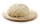The yeast cake contains milk, yeast, sugar, and flour in a ratio of 20: 4: 1: 15. What percentage of flour is in yeast?
• Red ballsIn the bag there are 3 red, 12 blue and 8 green balls. How many red balls we must be attached to the bag if we want the probability of pulling out the red balls was 20%?
• Siblings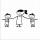Three siblings had saved up a total of 1,274 CZK. Peter had saved up to 15% more than Jirka and Hanka 10% less than Peter. How much money did they save each one of them?In six baskets, the seller has fruit. In individual baskets, there are only apples or just pears with the following number of fruits: 5,6,12,14,23 and 29. "If I sell this basket," the salesman thinks, "then I will have just as many apples as a pear." Whic
• DicesWe will throw two dice. What is the probability that the ratio between numbers on first and second dice will be 1:2?
• Event probability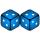The probability of event P in 8 independent experiments is 0.33. What is the probability that the event P occurs in one experiment (chance is the same)?
• Repair pipe20 workers had to repair broken pipes in 30 days. After fourteen days, four laborers joined them. How long did the pipe repair work last?
• In the orchardIn the orchard, they planted 25 apple trees, 20 pears, 15 plums and 40 marbles. A strong late frost, however, destroyed a fifth of all new trees. Unfortunately, it was all the trees of one kind of fruit. What is the probability that the plums have died ou
• Mushrooms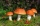The mushroom picker had 110 mushrooms in the basket. He had 36 mushrooms less than cossacks. How many mushrooms did he bring if he only had cossacks and mushrooms in the basket?
• Double probabilityThe probability of success of the planned action is 60%. What is the probability that we will achieve success at least once if this action is repeated twice?
• Football seasonDalibor and Adam together scored 97 goals in the season. Adam scored 9 goals more than Dalibor. How many goals scored each?
• Reward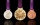Janko and Peter divided the reward from the brigade so that Peter got 5/8 of the reward. What percentage of Janko's reward got?
• Negative percentage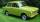In 2006, the company had a loss of 11100 Euros. Two years later, he was already in profit 50000 Eur. Calculate what percentage of the company increased profits in these two years.
• EurosPeter, Jane, and Thomas have together € 550. Tomas has 20 euros more than Jane, Peter € 150 less than Thomas. Determine how much has each of them.